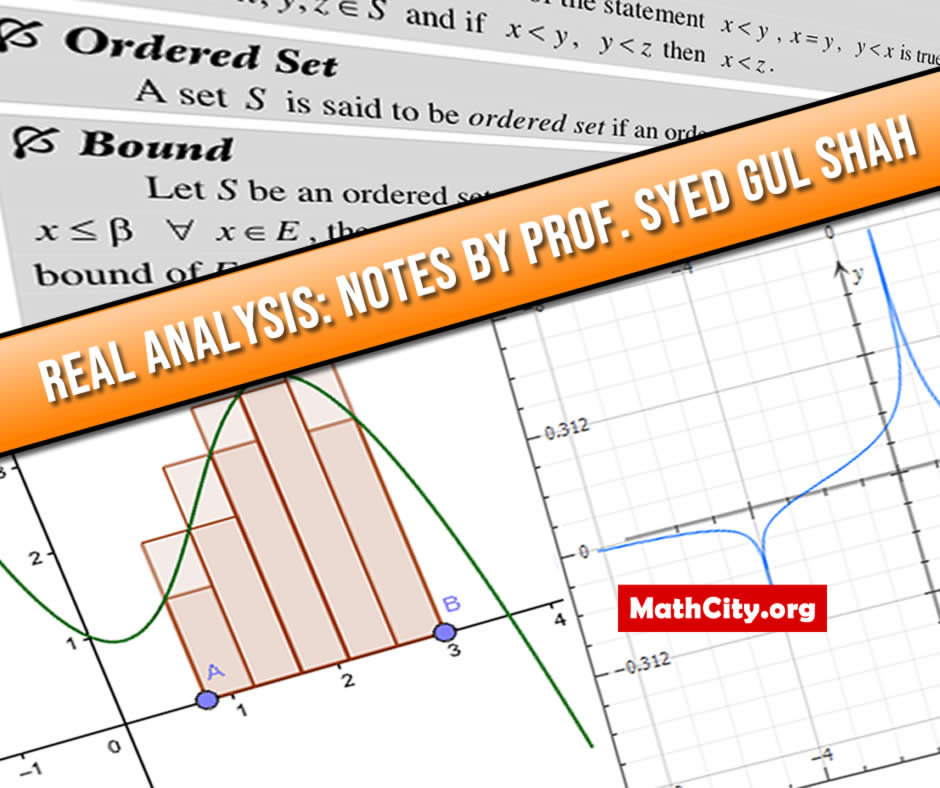# Real Analysis Notes by Prof Syed Gul ShahReal analysis, a discipline that explores the complexities of mathematical functions, limits, and sequences, can often be a difficult topic for students. Prof. Syed Gul Shah, as a true analyst, not only excelled in the subject but also gained fame for his extraordinary qualities as a human being.

As a teacher, Prof. Syed Gul Shah's dedication to excellence is unwavering. He goes above and beyond to ensure that his students thoroughly understand the material. He breaks down complex concepts into manageable chunks, patiently guiding his students through the intricacies of real-world analysis. His passion for the subject is palpable, and his enthusiasm is infectious. He instills in his students a deep appreciation for the beauty and elegance of mathematical analysis, inspiring them to pursue a lifelong love of the subject.

Apart from his exceptional teaching skills, Prof. Syed Gul Shah acts as a mentor and guide to his students. He encourages them to strive for excellence, believe in their abilities, and embrace challenges as opportunities for growth. He promotes a growth mindset, instilling in his students the belief that they can overcome any obstacle with persistence and determination.

Finally, Prof. Syed Gul Shah is a veritable tower of inspiration in the field of real analysis. His extraordinary qualities as a human being, his unwavering dedication to teaching, and his outstanding subject matter expertise make him a truly gifted educator. Her kindness, humility, and wisdom have a profound effect on her students, making them analytical thinkers and lifelong learners. Prof. Syed Gul Shah's legacy as a distinguished educator will undoubtedly endure for generations to come, and his contributions to the field of real analysis are numerous.

• Name: Real Analysis Notes by Prof Syed Gul Shah
• Author: Porf. Syed Gul Shah
• Pages: 176 pages
• Format: PDF
• Size: 1.75 MB
• Category: Analysis
• Level: MSc, BS Mathematics
• Chapter 01: Real Number system
• Chapter 02: Sequence and Series
• Sequence, Subsequence, Increasing Sequence, Decreasing Sequence, Monotonic Sequence, Strictly Increasing or Decreasing
• Bernoulli’s Inequality
• Bounded Sequence
• Convergence of the Sequence
• Theorem: A convergent sequence of real number has one and only one limit (i.e. limit of the sequence is unique.)
• Cauchy Sequence
• Theorem: A Cauchy sequence of real numbers is bounded.
• Divergent Sequence
• Theorem: If $s_n<u_n<t_n$ for all $n\ge n_0$ and if both the $\{s_n\}$ and $\{t_n\}$ converge to same limits as s, then the sequence $\{u_n\}$ also converges to s.
• Theorem: If the sequence $\{s_n\}$ converges to s then $\exists$ a positive integer such that $\left| {\,{s_n}}\right|>\frac{1}{2}s$.
• Theorem: Let a and b be fixed real numbers if $\{s_n\}$ and $\{t_n\}$ converge to s and t respectively. Then (i) $\left\{a{s_n}+b{t_n}\right\}$ converges to $as+bt$. (ii) $\left\{{s_n}{t_n}\right\}$ converges to st. (iii) $\left\{\frac{{{s_n}}}{{{t_n}}} \right\}$ converges to $\frac{s}{t}$ provided ${t_n}\ne 0\,\,\,\,\forall \,\,\,n$ and $t\ne 0$.
• Theorem: For each irrational number x, there exists a sequence $\left\{{r_n}\right\}$ of distinct rational numbers such that $\lim_{n\to \infty}{r_n}=x$.
• Let a sequence $\{s_n\}$ be a bounded sequence. (i) If $\{s_n\}$ is monotonically increasing then it converges to its supremum. (ii) If $\{s_n\}$ is monotonically decreasing then it converges to its infimum.
• Recurrence Relation
• Theorem: Every Cauchy sequence of real numbers has a convergent subsequence.
• Theorem (Cauchy’s General Principle for Convergence): A sequence of real number is convergent if and only if it is a Cauchy sequence.
• Theorem (nested intervals): Suppose that $\{I_n}\}$ is a sequence of the closed interval such that ${I_n} = \left[a_n,b_n\right]$, $I_{n + 1}\subset I_n \,\,\,\forall \,\,\,n\ge 1$ and $(b_n-a_n)\to 0$ as $n\to \infty$ then $\cap {I_n}$ contains one and only one point.
• Theorem (Bolzano-Weierstrass theorem): Every bounded sequence has a convergent subsequence.
• Limit inferior of the sequence.
• Limit superior of the sequence.
• Theorem: If $\{s_n\}$ is a convergent sequence then $\lim_{n\to\infty} {s_n}=\lim_{n\to\infty} \left(\inf{s_n} \right)=\lim_{n\to\infty}\left(\sup {s_n}\right)$.
• Infinite Series.
• Theorem: If $\sum_{n=1}^\infty{a_n}$ converges then $\lim_{n\to\infty} {a_n}=0$.
• Theorem (General Principle of Convergence): A series $\sum{a_n}$ is convergent if and only if for any real number $\varepsilon >0$, there exists a positive integer $n_0$ such that $\left|\sum_{i=m+1}^\infty {a_i}\right|< \varepsilon$ for all $n>m>n_0$.
• Theorem: Let $\sum {a_n}$ be an infinite series of non-negative terms and let $\{s_n\}$ be a sequence of its partial sums then $\sum{a_n}$ is convergent if $\{s_n\}$ is bounded and it diverges if $\{s_n\}$ is unbounded.
• Theorem (Comparison Test)
• Theorem: Let $a_n>0, b_n>0$ and $\lim_{n\to\infty } \frac{a_n}{b_n}=\lambda\ne 0$ then the series $\sum{a_n}$ and $\sum{b_n}$ behave alike.
• Theorem ( Cauchy Condensation Test )
• Alternating Series.
• Theorem (Alternating Series Test or Leibniz Test)
• Absolute Convergence
• Theorem: An absolutely convergent series is convergent.
• Theorem (The Root Test)
• Theorem (Ratio Test)
• Theorem (Dirichlet): Suppose that $\{s_n}\}, {s_n}={a_1}+{a_2}+{a_3}+...+ {a_n}$ is bounded. Let $\{b_n\}$ be positive term decreasing sequence such that $\lim_{n\to\infty}{b_n}=0$. Then $\sum{a_n b_n}$ is convergent.
• Theorem: Suppose that $\sum{a_n}$ is convergent and that $\{b_n\}$ is monotonic convergent sequence then $\sum{a_n b_n}$ is also convergent.
• Lot of examples.
• Chapter 03: Limits and Continuity
• Chapter 04: Differentiation
• Chapter 05: Functions of Several Variables
• Examples and Question about “Maxima and Minima of Function of Two Variable”
• Examples and Question about “Maxima and Minima of Function of Two or More Variable with Side Conditions, Lagrange's Multiplier”
• Chapter 06: Riemann-Stieltjes I ntegral
• Chapter 07: Functions of Bounded Variation
• Chapter 08: Improper Integrals

• notes/real-analysis-notes-by-prof-syed-gul-shah